Home | ARTS | Operations Management | Solve a game by simplex method - Linear Programming Approach To Game Theory

# Solve a game by simplex method - Linear Programming Approach To Game Theory

Posted On :  25.06.2018 02:00 am

Solve the following game by simplex method for LPP:

Solve a game by simplex method

### Problem 1

Solve the following game by simplex method for LPP: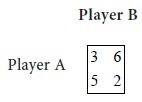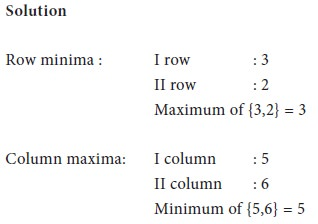So, Maximum of {Row minima}   Minimum of {Column maxima}.

Therefore the given game has no saddle point.  It is a mixed game.

Let us convert the given game into a LPP.

### Problem formulation

Let V denote the value of the game. Let the probability that the player B will use his first strategy be r and second strategy be s. Let V denote the value of the game.

When A follows his first strategy

The expected payoff to A (i.e., the expected loss to B) = 3 r + 6 s.

This pay-off cannot exceed V. So we have  =3 r + 6 s V        (1)

When A follows his second strategy

The expected pay-off to A (i.e., expected loss to B) = 5 r + 2 s.

This cannot exceed V. Hence we obtain the condition = 5 r + 2 s V        (2)

From (1) and (2) we have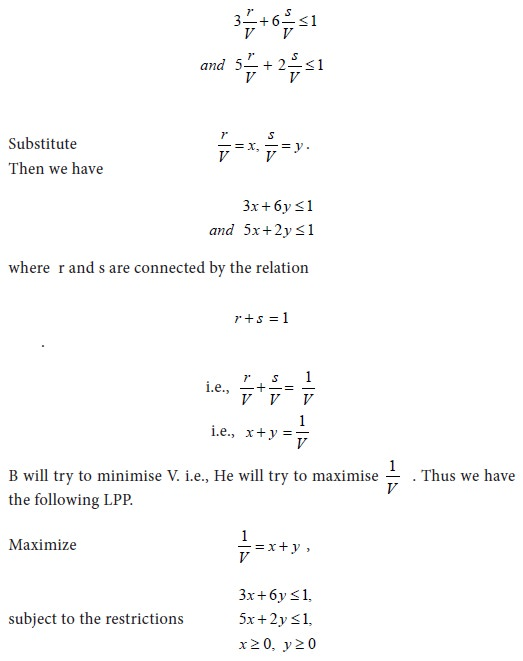### Solution of LPPConsider the negative elements in the objective function row. They are –1, -1. The absolute values are 1, 1. There is a tie between these coefficients. To resolve the tie, we select the variable x. We take the new basic variable as x. Consider the ratio of b-value to x-value. We have the following ratios: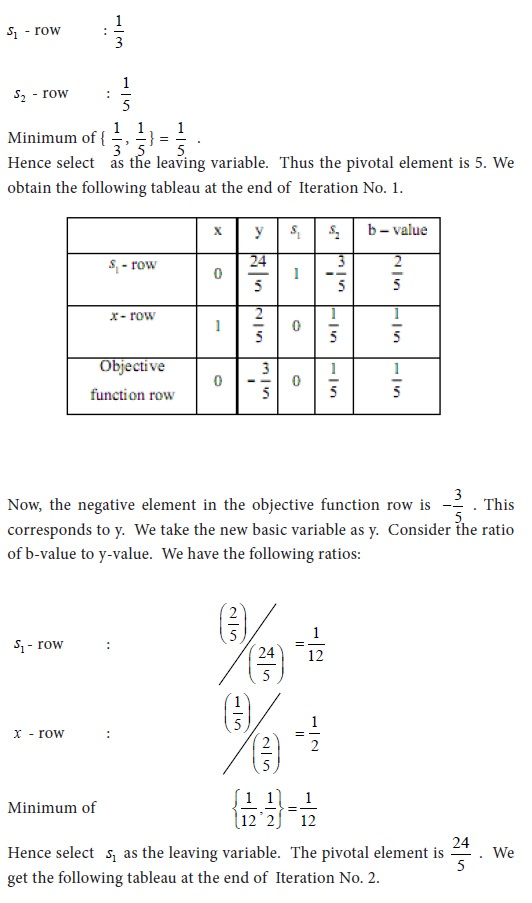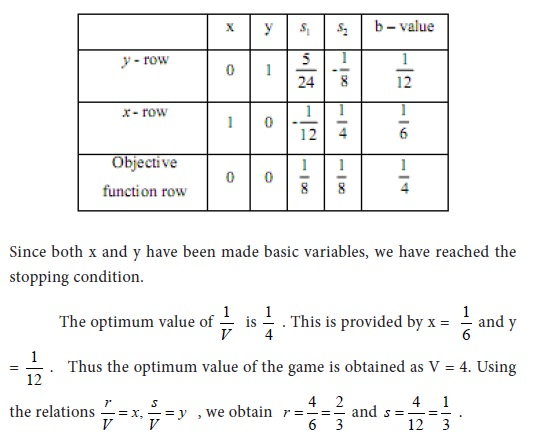### Problem 2

Solve the following game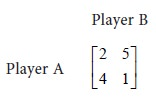Solution

The game has no saddle point. It is a mixed game. Let the probability that B will use his first strategy be r. Let the probability that B will use his second strategy be s. Let V be the value of the game.

When A follows his first strategy

The expected payoff to A (i.e., the expected loss to B) = 2 r +5 s.

The pay-off to A cannot exceed V. So we have       = 2 r + 5 s V          (I)

When A follows his second strategy

The expected pay-off to A (i.e., expected loss to B) = 4 r + s.

The pay-off to A cannot exceed V. Hence we obtain the condition = 4 r + s V (II)

From (I) and (II) we have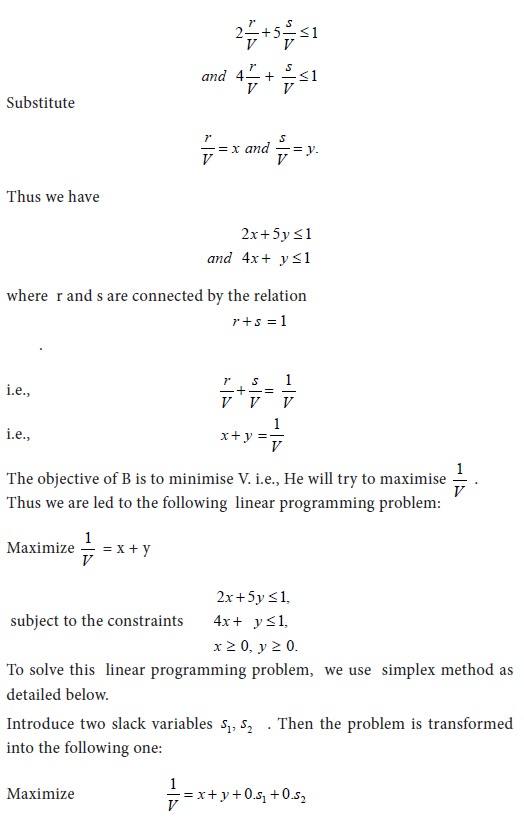Consider the negative elements in the objective function row. They are –1, -1. The absolute value are 1, 1. There is a tie between these coefficients. To resolve the tie, we select the variable x. We take the new basic variable as x. Consider the ratio of b-value to x-value. We have the following ratios: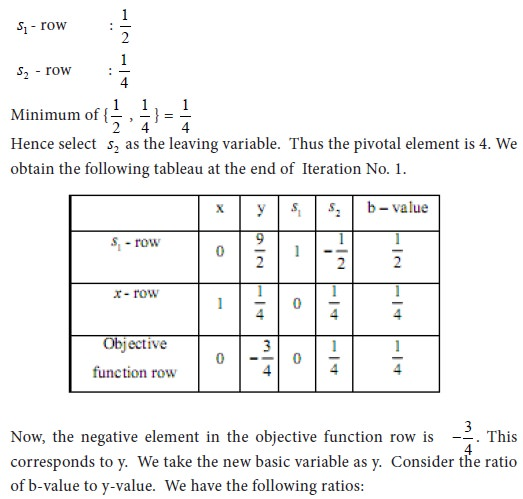## Problem 3

Solve the following game by simplex method for LPP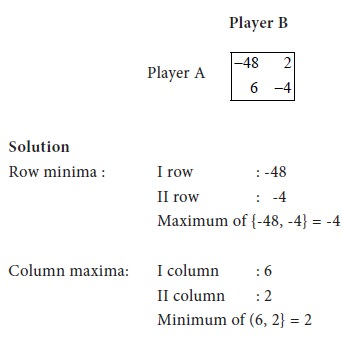So, Maximum of {Row minima}   Minimum of {Column maxima}.

Therefore the given game has no saddle point.  It is a mixed game. Let us convert the given game into a LPP.

### Problem formulation

Let V denote the value of the game. Let the probability that the player B will use his first strategy be r and second strategy be s. Let V denote the value of the game.

 When A follows his first strategy The expected payoff to A (i.e., the expected loss to B) = - 48 r + 2 s. This pay-off cannot exceed V. So we have =- 48 r + 2 s V (1)’

When A follows his second strategy

The expected pay-off to A (i.e., expected loss to B) = 6 r - 4 s.

This cannot exceed V. Hence we obtain the condition = 6 r - 4 s V (2)’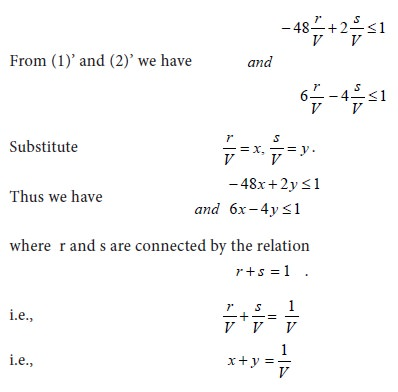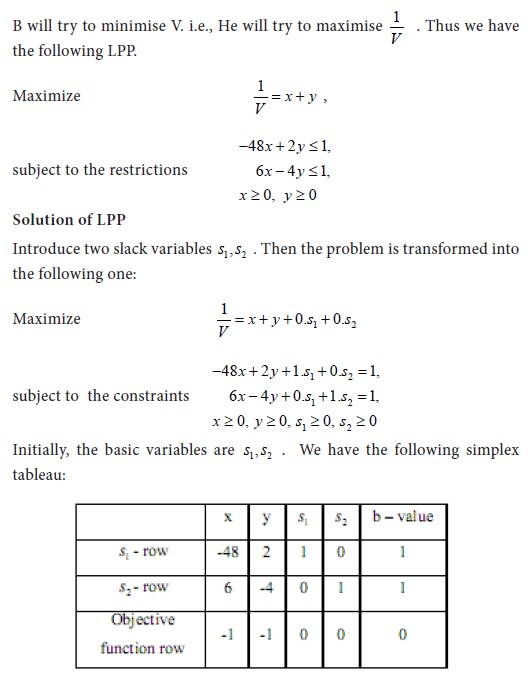Consider the negative elements in the objective function row. They are –1, -1. The absolute value are 1, 1. There is a tie between these coefficients. To resolve the tie, we select the variable x. We take the new basic variable as x. Consider the ratio of b-value to x-value. We have the following ratios:## Problem 4

Transform the following game into an LPP

s = 9/10

Solution

We have to determine the optimal strategy for player B. Using the entries of the given matrix, we obtain the inequalitiesTags : Operations Management - Game Theory, Goal Programming & Queuing Theory
Last 30 days 326 views